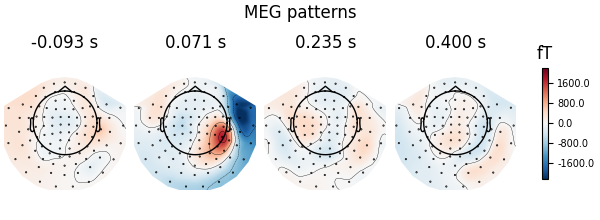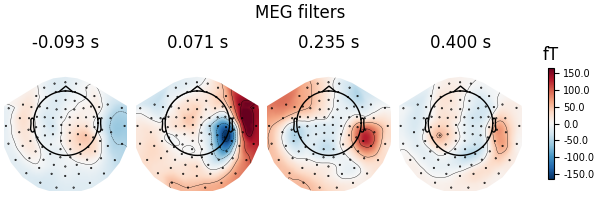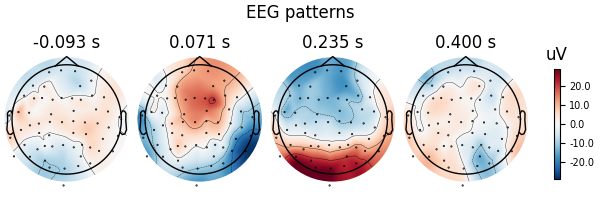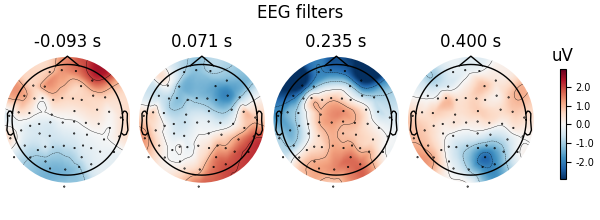# Linear classifier on sensor data with plot patterns and filters#

Here decoding, a.k.a MVPA or supervised machine learning, is applied to M/EEG data in sensor space. Fit a linear classifier with the LinearModel object providing topographical patterns which are more neurophysiologically interpretable 1 than the classifier filters (weight vectors). The patterns explain how the MEG and EEG data were generated from the discriminant neural sources which are extracted by the filters. Note patterns/filters in MEG data are more similar than EEG data because the noise is less spatially correlated in MEG than EEG.

```# Authors: Alexandre Gramfort <alexandre.gramfort@inria.fr>
#          Romain Trachel <trachelr@gmail.com>
#          Jean-Remi King <jeanremi.king@gmail.com>
#
```
```import mne
from mne import io, EvokedArray
from mne.datasets import sample
from mne.decoding import Vectorizer, get_coef

from sklearn.preprocessing import StandardScaler
from sklearn.linear_model import LogisticRegression
from sklearn.pipeline import make_pipeline

# import a linear classifier from mne.decoding
from mne.decoding import LinearModel

print(__doc__)

data_path = sample.data_path()
sample_path = data_path / "MEG" / "sample"
```

Set parameters

```raw_fname = sample_path / "sample_audvis_filt-0-40_raw.fif"
event_fname = sample_path / "sample_audvis_filt-0-40_raw-eve.fif"
tmin, tmax = -0.1, 0.4
event_id = dict(aud_l=1, vis_l=3)

# Setup for reading the raw data
raw.filter(0.5, 25, fir_design="firwin")

epochs = mne.Epochs(
raw, events, event_id, tmin, tmax, proj=True, decim=2, baseline=None, preload=True
)
del raw

labels = epochs.events[:, -1]

# get MEG and EEG data
meg_epochs = epochs.copy().pick_types(meg=True, eeg=False)
meg_data = meg_epochs.get_data().reshape(len(labels), -1)
```
```Opening raw data file /home/circleci/mne_data/MNE-sample-data/MEG/sample/sample_audvis_filt-0-40_raw.fif...
Read a total of 4 projection items:
PCA-v1 (1 x 102)  idle
PCA-v2 (1 x 102)  idle
PCA-v3 (1 x 102)  idle
Average EEG reference (1 x 60)  idle
Range : 6450 ... 48149 =     42.956 ...   320.665 secs
Reading 0 ... 41699  =      0.000 ...   277.709 secs...
Filtering raw data in 1 contiguous segment
Setting up band-pass filter from 0.5 - 25 Hz

FIR filter parameters
---------------------
Designing a one-pass, zero-phase, non-causal bandpass filter:
- Windowed time-domain design (firwin) method
- Hamming window with 0.0194 passband ripple and 53 dB stopband attenuation
- Lower passband edge: 0.50
- Lower transition bandwidth: 0.50 Hz (-6 dB cutoff frequency: 0.25 Hz)
- Upper passband edge: 25.00 Hz
- Upper transition bandwidth: 6.25 Hz (-6 dB cutoff frequency: 28.12 Hz)
- Filter length: 993 samples (6.613 s)

[Parallel(n_jobs=1)]: Done  17 tasks      | elapsed:    0.0s
[Parallel(n_jobs=1)]: Done  71 tasks      | elapsed:    0.1s
[Parallel(n_jobs=1)]: Done 161 tasks      | elapsed:    0.2s
[Parallel(n_jobs=1)]: Done 287 tasks      | elapsed:    0.4s
145 matching events found
No baseline correction applied
Created an SSP operator (subspace dimension = 4)
4 projection items activated
Using data from preloaded Raw for 145 events and 76 original time points (prior to decimation) ...
NOTE: pick_types() is a legacy function. New code should use inst.pick(...).
Removing projector <Projection | Average EEG reference, active : True, n_channels : 60>
```

## Decoding in sensor space using a LogisticRegression classifier#

```clf = LogisticRegression(solver="liblinear")  # liblinear is faster than lbfgs
scaler = StandardScaler()

# create a linear model with LogisticRegression
model = LinearModel(clf)

# fit the classifier on MEG data
X = scaler.fit_transform(meg_data)
model.fit(X, labels)

# Extract and plot spatial filters and spatial patterns
for name, coef in (("patterns", model.patterns_), ("filters", model.filters_)):
# We fitted the linear model onto Z-scored data. To make the filters
# interpretable, we must reverse this normalization step
coef = scaler.inverse_transform([coef])

# The data was vectorized to fit a single model across all time points and
# all channels. We thus reshape it:
coef = coef.reshape(len(meg_epochs.ch_names), -1)

# Plot
evoked = EvokedArray(coef, meg_epochs.info, tmin=epochs.tmin)
fig = evoked.plot_topomap()
fig.suptitle(f"MEG {name}")
```
••Let’s do the same on EEG data using a scikit-learn pipeline

```X = epochs.pick_types(meg=False, eeg=True)
y = epochs.events[:, 2]

# Define a unique pipeline to sequentially:
clf = make_pipeline(
Vectorizer(),  # 1) vectorize across time and channels
StandardScaler(),  # 2) normalize features across trials
LinearModel(  # 3) fits a logistic regression
LogisticRegression(solver="liblinear")
),
)
clf.fit(X, y)

# Extract and plot patterns and filters
for name in ("patterns_", "filters_"):
# The `inverse_transform` parameter will call this method on any estimator
# contained in the pipeline, in reverse order.
coef = get_coef(clf, name, inverse_transform=True)
evoked = EvokedArray(coef, epochs.info, tmin=epochs.tmin)
fig = evoked.plot_topomap()
fig.suptitle(f"EEG {name[:-1]}")
```
••```NOTE: pick_types() is a legacy function. New code should use inst.pick(...).
Removing projector <Projection | PCA-v1, active : True, n_channels : 102>
Removing projector <Projection | PCA-v2, active : True, n_channels : 102>
Removing projector <Projection | PCA-v3, active : True, n_channels : 102>
```